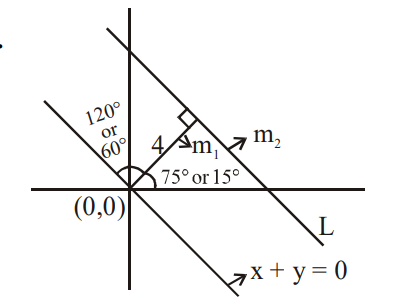Deepak Scored 45->99%ile with Bounce Back Crack Course. You can do it too!

# A straight line L at a distance of 4 units from the origin

Question:

A straight line $\mathrm{L}$ at a distance of 4 units from the origin makes positive intercepts on the coordinate axes and the perpendicular from the origin to this line makes an angle of $60^{\circ}$ with the line $\mathrm{x}+\mathrm{y}=0$. Then an equation of the line $\mathrm{L}$ is :

1. $(\sqrt{3}+1) \mathrm{x}+(\sqrt{3}-1) \mathrm{y}=8 \sqrt{2}$

2. $(\sqrt{3}-1) x+(\sqrt{3}+1) y=8 \sqrt{2}$

3. $\sqrt{3} x+y=8$

4. $x+\sqrt{3} y=8$

Correct Option: 1

Solution:$\mathrm{m}_{1}=\tan 75^{\circ}=\frac{\sqrt{3}+1}{\sqrt{3}-1}$

or $m=\tan 15^{\circ}=\frac{\sqrt{3}-1}{\sqrt{3}+1}$

$\mathrm{m}_{2}=\frac{-1}{\mathrm{~m}_{1}}=\frac{-(\sqrt{3}-1)}{\sqrt{3}+1}$

or $\mathrm{m}_{2}=\frac{-1}{\mathrm{~m}_{1}}=\frac{-(\sqrt{3}+1)}{\sqrt{3}-1}$

$\Rightarrow \mathrm{y}=\mathrm{m}_{2} \mathrm{x}+\mathrm{C} \Rightarrow \mathrm{y}=\frac{-(\sqrt{3}-1) \mathrm{x}}{\sqrt{3}+1}+\mathrm{C} \Rightarrow \mathrm{L}$

or $\mathrm{y}=\frac{-(\sqrt{3}+1) \mathrm{x}}{\sqrt{3}-1}+\mathrm{C} \Rightarrow \mathrm{L}$

Distance from origin $=4$

$\therefore\left|\frac{\mathrm{C}}{\sqrt{1+\frac{(\sqrt{3}-1)^{2}}{(\sqrt{3}+1)^{2}}}}\right|=4$ or $\left|\frac{\mathrm{C}}{\sqrt{1+\frac{(\sqrt{3}+1)^{2}}{(\sqrt{3}-1)^{2}}}}\right|=4$

$\Rightarrow \mathrm{C}=\frac{8 \sqrt{2}}{(\sqrt{3}+1)}$ or $\mathrm{C}=\frac{8 \sqrt{2}}{(\sqrt{3}-1)}$

$\Rightarrow(\sqrt{3}-1) y+(\sqrt{3}+1) x=8 \sqrt{2}$

or $(\sqrt{3}-1) x+(\sqrt{3}+1) y=8 \sqrt{2}$